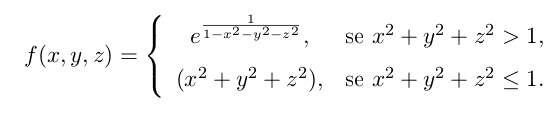# Limit of a function of two variables

Jalo

## Homework Statement

Given the functionStudy its continuity

## The Attempt at a Solution

I don't know how to solve this function. Normally I'd try to prove the limit doesn't exist by getting different results trough different ways. However, in this function I don't have a point where the continuity might or might not exist. I have a conjunct of points.

Can anyone give me a hint on how to solve this?

## Answers and Replies

Homework Helper
You should have, if you are given an exercise like this, know the theorem that says "a function of two variables is continuous at a point if and only if it is continuous in each variable separately at that point". And even before that you should have learned that elementary functions are continuous where ever the are defined.

Here, all functions are "elementary" and the only place the function is undefined is where a denominator is 0: where $1- x^2- y^2- z^2= 0$- i.e. on the surface of the unit sphere. Now, what is the limit as you approach the surface of the sphere from "inside" and from "outside"?

Jalo
You should have, if you are given an exercise like this, know the theorem that says "a function of two variables is continuous at a point if and only if it is continuous in each variable separately at that point". And even before that you should have learned that elementary functions are continuous where ever the are defined.

Here, all functions are "elementary" and the only place the function is undefined is where a denominator is 0: where $1- x^2- y^2- z^2= 0$- i.e. on the surface of the unit sphere. Now, what is the limit as you approach the surface of the sphere from "inside" and from "outside"?

z=x²+y²+z²)

limz→1 e1/(1-z) = e1/0 = e = ∞ ≠ (f(0,0) = 1)

Is this resolution correct?